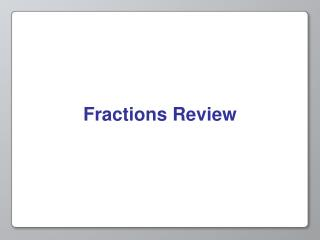DownloadDownload PresentationFractions Review

# Fractions Review

Télécharger la présentation## Fractions Review

- - - - - - - - - - - - - - - - - - - - - - - - - - - E N D - - - - - - - - - - - - - - - - - - - - - - - - - - -
##### Presentation Transcript

1. Fractions Review

2. Fractions A number in the form Numerator Denominator Or N D

3. Fractions The denominator can never be equal to 0. 12 Does not exist! = 0

4. Fractions A fraction with a numerator of 0 equals 0. 0 0 = 0 = 0 4 156

5. Fractions • If the numerator is larger than the denominator, it is called an improper fraction. Find the improper fraction 7 10 4 56 10 11 8 9 73 12

6. Math with Fractions Four basic functions • Multiply • Divide • Add • Subtract

7. Multiplication • Multiply the numerators and put in the numerator of the result • Multiply the denominators and put in the denominator of the result 7 4 7 x 4 28 x = = 8 9 8 x 9 72

8. Multiplication - Let’s Try It! 7 1 7 4 9 36 x x = = 9 2 18 7 11 77 30 7 7 1 7 210 x x = = 4 14 5 3 15 56

9. These numbers get pretty big! 210 56 What if we needed to multiply again? Let’s make the fraction more simple, so it will be easier to use in the future.

10. Simplification • Divide by the Greatest Common Factor 28 But what is a Common Factor? 72

11. Factors • A factor is a number that can be divided into another number with no remainder • 8’s factors are: • 1 (8/1 =8) • 2 (8/2 = 4) • 4 (8/4 = 2) • 8 (8/8 = 1) • 3 is NOT a factor of 8, because 8 is not evenly divisible by 3 (8/3 = 2 with R=3)

12. Common Factors • A common factor is a factor that two numbers have in common • For example, 7 is a factor of both 21 and 105, so it is a common factor of the two. • The greatest common factor is the largest factor that the two number share So let’s go back to our simplification problem from before…

13. Simplification • Divide both numerator and denominator by the Greatest Common Factor 28 Factors are 1, 2, 4, 7, 14, 28 Factors are 1, 2, 3, 4, 6, 8, 9, 12, 18, 24, 48, 72 72 Greatest Common Factor is 4 28 7 ÷ = 28 4 7 = So = 72 18 72 ÷ 4 18

14. Simplification - Let’s Try It! 3 1 7 1 18 2 = = = 9 3 21 3 63 7 24 2 6 2 78 13 = = = 84 7 15 5 114 19

15. Division • Just like multiplication with one more step • Invert the second fraction and multiply 6 3 1 3 2 x ÷ = = 8 8 2 8 1

16. Division - Let’s Try It! 7 1 14 4 9 44 ÷ = ÷ = 9 2 9 7 11 63 7 1 21 20 7 50 ÷ = ÷ = 5 3 5 4 10 7

17. Addition • To add two fractions, you must make sure they have a Common Denominator What is a Common Denominator? 3 5 + 8 16

18. Common Denominator • A common denominator is a number with which both of the denominators share at least one factor that is not the number 1 • For example, if the denominators are 4 and 7, then a common denominator is 28. • 28 shares the factors 1, 2 and 4 with the number 4, and the factors 1 and 7 with the number 7. So let’s go back to our simplification problem from before…

19. 3 2 x = 8 2 Addition • To add two fractions, you must make sure they have a Common Denominator • What can you multiply each fraction by to give the smallest common denominator? 3 5 16 goes into 16 one time 8 goes into 16 two times + 5 1 5 6 x = 8 16 16 1 16 16 The smallest number that has both of these as factors is 16 6 5 11 + = 16 16 16 Once you have a common denominator, add the numerators.

20. Addition - Let’s Try It! 1 1 3 4 2 1 + = + = 4 2 4 16 8 2 6 2 17 13 3 25 + = + = 8 3 12 16 4 16

21. 3 2 6 5 1 5 x x = = 8 2 16 16 1 16 Subtraction • To subtract two fractions, they also must have a Common Denominator • What can you multiply each fraction by to give the smallest common denominator? 3 5 8 goes into 16 two times 16 goes into 16 one time - 8 16 6 5 1 The smallest number that has both of these as factors is 16 - = 16 16 16 Once you have a common denominator, subtract the numerators.

22. Subtraction - Let’s Try It! 7 1 3 9 3 3 - - = = 8 2 8 16 8 16 6 1 1 5 7 13 - - = = 8 2 4 4 16 16

23. Review • A fraction has a numerator and a denominator • The denominator can never be 0 • You can multiply, divide, add and subtract fractions • A common factor is a number that both denominators are evenly divisible by • A common denominator is a number that both denominators share a factor with